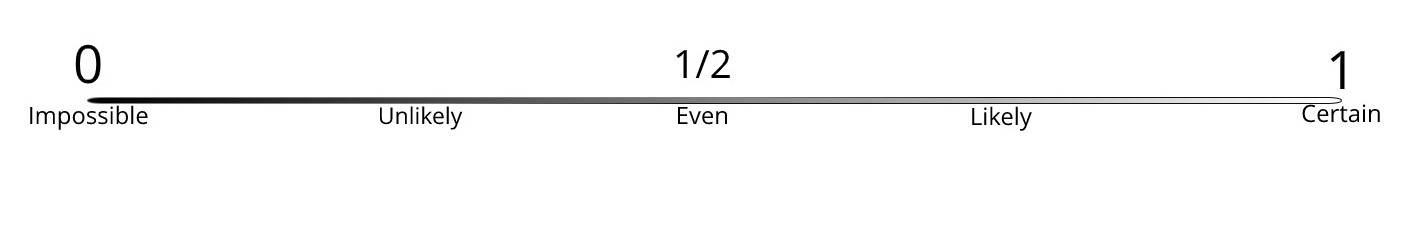# Summary 1

Last updated: March 10th, 2020

# Summary 1¶

So far we have seen that we can perform experiments, which are procedures that can be repeated.

We've said that these experiments can be classified into:

• deterministic experiments: those that have a result completely determined,
• random experiments: those that have results subject to chance.

We've called outcome to every result we get when we perform an experiment, and we've organised them in a set called sample space.

The sample space is denoted with the Greek capital letter Omega $\Omega$, and every outcome in the sample space is denoted with the Greek lower case omega $\omega$.

Mathematically we write $\omega\in\Omega$.

An interesting thing we've noticed is that the sample space of any deterministic experiment has only one element, which is the only possible outcome of the experiment.

After that, we've defined two different types of probabilities:

• theoretical probability: which is the likelihood of an outcome, mathematically written $P(\omega)$ for $\omega\in\Omega$,
• empirical probability: which is the relative frequency of an outcome, or the proportion of times we get that outcome.

We've explored some empirical probabilities and found that the more times we perform an experiment, the closer that gets to the theoretical probability.

At this point, we established that the probability of an impossible outcome is $0$, and the probability of a certain outcome is $1$.

With this, we realized that every probability will be between $0$ and $1$. Mathematically, for any $\omega\in\Omega$, $0\leq P(\omega)\leq 1$.We then defined the concept of event as any subset of the sample space, and realised that we can use set operations to describe more complex events.

Also, we discovered that $P(\omega)=P(\{\omega\})$, which will allow us to think only in terms of probabilities of events instead of probabilities of outcomes.

We then introduced two very special events:

• $\emptyset$: which is the event of nothing happening,
• $\Omega$: which is the event of anything happening

Again, we established that $P(\emptyset)=0$ and $P(\Omega)=1$.

Finally, we've learnt that the probabilities of events that make the whole sample space add up to $1$.